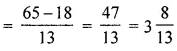# ML Aggarwal Class 6 Solutions for ICSE Maths Chapter 6 Fractions Ex 6.5

## ML Aggarwal Class 6 Solutions for ICSE Maths Chapter 6 Fractions Ex 6.5

Question 1.
Work out the following :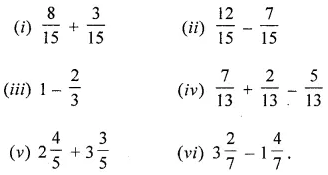Solution: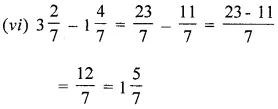Question 2.
Find in the missing fractions:Solution: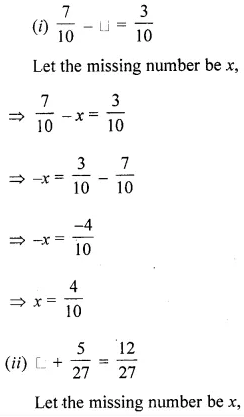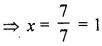Question 3.
Work out the following:Solution: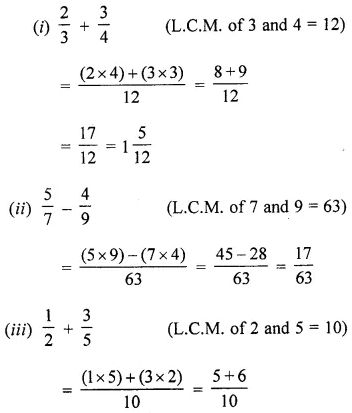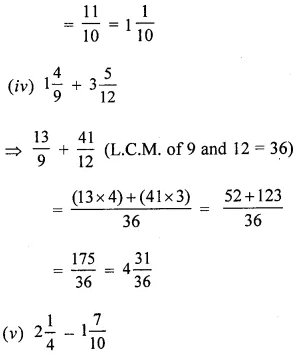Question 4.
Simplify the following:Solution: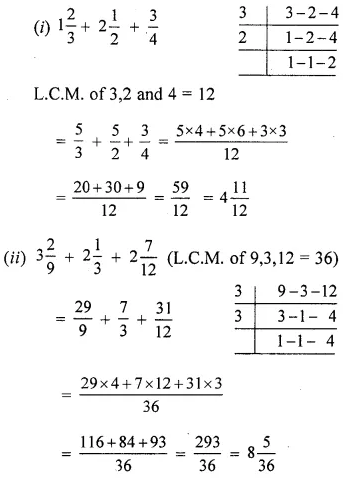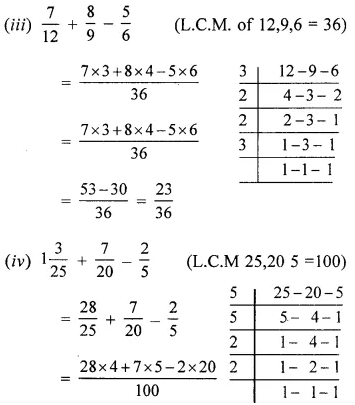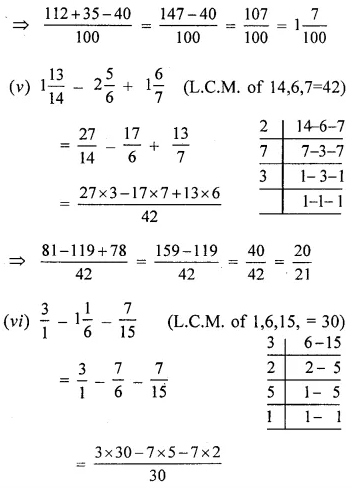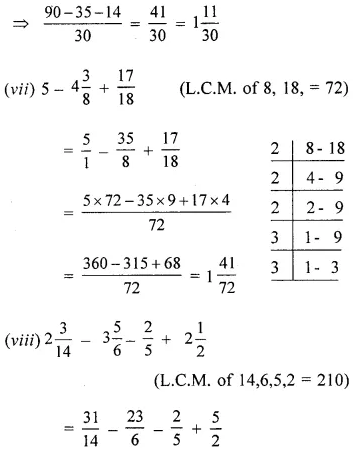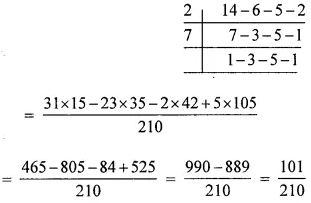Question 5.
(i) What number should be added to $$\frac{5}{12}$$ to get $$2 \frac{3}{8}$$?
(ii) What number should be subtracted from 5 to get $$1 \frac{5}{13}$$ ?
Solution: